# The Bootstrap and Consonance Functions

Some authors have shown that the bootstrap distribution is equal to the confidence distribution because it meets the definition of a consonance distribution.13 The bootstrap distribution and the asymptotic consonance distribution would be defined as:

$H_{n}(\theta)=1-P\left(\hat{\theta}-\hat{\theta}^{*} \leq \hat{\theta}-\theta | \mathbf{x}\right)=P\left(\hat{\theta}^{*} \leq \theta | \mathbf{x}\right)$

Certain bootstrap methods such as the BCa method and t-bootstrap method also yield second order accuracy of consonance distributions.

$H_{n}(\theta)=1-P\left(\frac{\hat{\theta}^{*}-\hat{\theta}}{\widehat{S E}^{*}\left(\hat{\theta}^{*}\right)} \leq \frac{\hat{\theta}-\theta}{\widehat{S E}(\hat{\theta})} | \mathbf{x}\right)$

Here, I demonstrate how to use these particular bootstrap methods to arrive at consonance curves and densities.

We’ll use the Iris dataset and construct a function that’ll yield a parameter of interest.

## The Nonparametric Bootstrap

iris <- datasets::iris
foo <- function(data, indices) {
dt <- data[indices, ]
c(
cor(dt[, 1], dt[, 2], method = "p")
)
}

We can now use the curve_boot() method to construct a function. The default method used for this function is the “Bca” method provided by the bcaboot package.2

I will suppress the output of the function because it is unnecessarily long. But we’ve placed all the estimates into a list object called y.

The first item in the list will be the consonance distribution constructed by typical means, while the third item will be the bootstrap approximation to the consonance distribution.

ggcurve(data = y[], nullvalue = TRUE)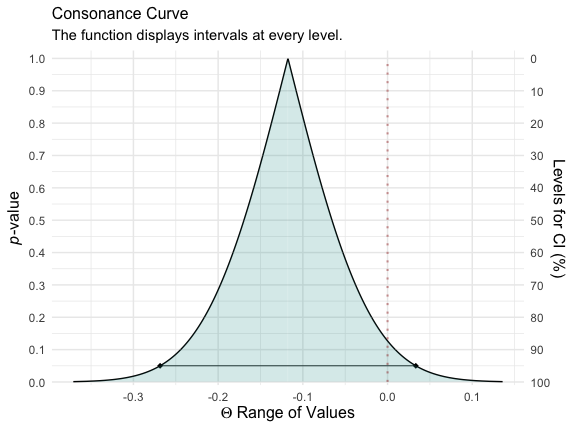ggcurve(data = y[], nullvalue = TRUE)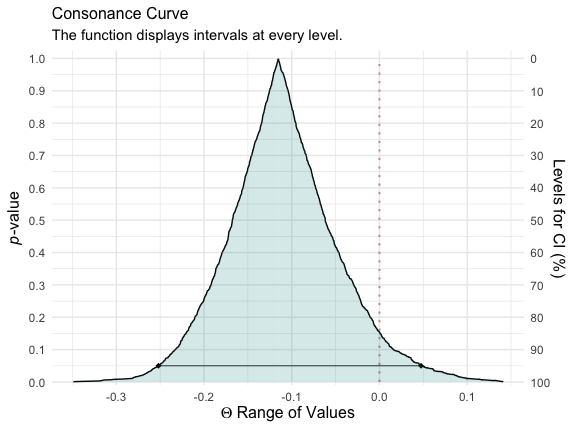We can also print out a table for TeX documents

(gg <- curve_table(data = y[], format = "image"))
 Lower Limit Upper Limit Interval Width Interval Level (%) CDF P-value S-value (bits) -0.142 -0.093 0.049 25 0.625 0.75 0.415 -0.170 -0.066 0.104 50 0.750 0.50 1.000 -0.206 -0.029 0.177 75 0.875 0.25 2.000 -0.216 -0.019 0.197 80 0.900 0.20 2.322 -0.268 0.033 0.302 95 0.975 0.05 4.322 -0.316 0.081 0.397 99 0.995 0.01 6.644

More bootstrap replications will lead to a smoother function. But for now, we can compare these two functions to see how similar they are.

plot_compare(y[], y[])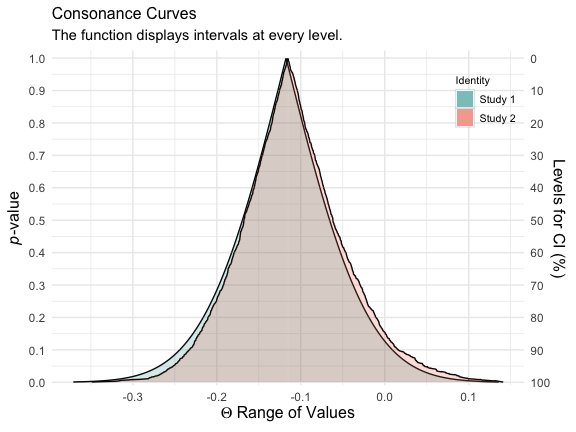If we wanted to look at the bootstrap standard errors, we could do so by loading the fifth item in the list

knitr::kable(y[])
theta sdboot z0 a sdjack
est -0.1175698 0.0770054 0.0313380 0.0304863 0.075694
jsd 0.0000000 0.0011757 0.0234461 0.0000000 0.000000

where in the top row, theta is the point estimate, and sdboot is the bootstrap estimate of the standard error, sdjack is the jacknife estimate of the standard error. z0 is the bias correction value and a is the acceleration constant.

The values in the second row are essentially the internal standard errors of the estimates in the top row.

The densities can also be calculated accurately using the t-bootstrap method. Here we use a different dataset to show this

library(Lock5Data)
dataz <- data(CommuteAtlanta)
func <- function(data, index) {
x <- as.numeric(unlist(data))
y <- as.numeric(unlist(data))
return(mean(x[index]) - mean(y[index]))
}

Our function is a simple mean difference. This time, we’ll set the method to “t” for the t-bootstrap method

z <- curve_boot(data = CommuteAtlanta, func = func, method = "t", replicates = 2000, steps = 1000)
#> Warning in norm.inter(t, alpha): extreme order statistics used as endpoints
ggcurve(data = z[], nullvalue = FALSE)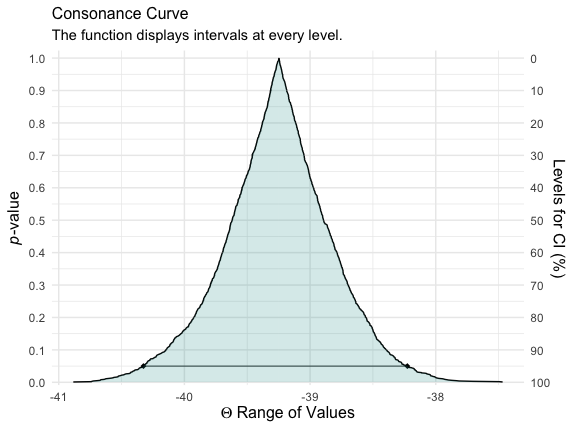ggcurve(data = z[], type = "cd", nullvalue = FALSE)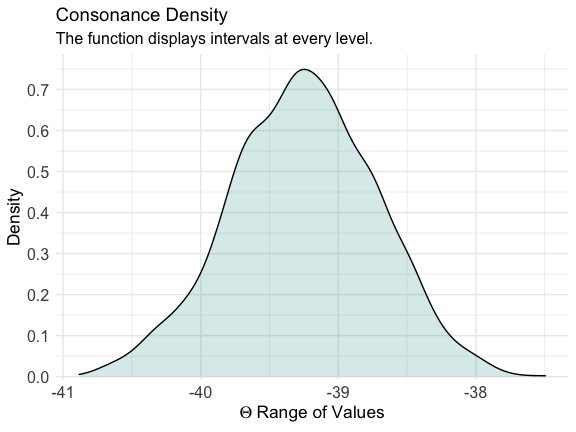The consonance curve and density are nearly identical. With more bootstrap replications, they are very likely to converge.

(zz <- curve_table(data = z[], format = "image"))
 Lower Limit Upper Limit Interval Width Interval Level (%) CDF P-value S-value (bits) -39.417 -39.078 0.339 25.0 0.625 0.750 0.415 -39.619 -38.893 0.727 50.0 0.750 0.500 1.000 -39.852 -38.638 1.214 75.0 0.875 0.250 2.000 -39.924 -38.555 1.369 80.0 0.900 0.200 2.322 -40.028 -38.488 1.540 85.0 0.925 0.150 2.737 -40.146 -38.394 1.752 90.0 0.950 0.100 3.322 -40.326 -38.226 2.100 95.0 0.975 0.050 4.322 -40.472 -38.062 2.410 97.5 0.988 0.025 5.322 -40.630 -37.936 2.694 99.0 0.995 0.010 6.644

## The Parametric Bootstrap

For the examples above, we mainly used nonparametric bootstrap methods. Here I show an example using the parametric Bca bootstrap and the results it yields.

First, we’ll load our data again and set our function.

data(diabetes, package = "bcaboot")
X <- diabetes$x y <- scale(diabetes$y, center = TRUE, scale = FALSE)
lm.model <- lm(y ~ X - 1)
mu.hat <- lm.model$fitted.values sigma.hat <- stats::sd(lm.model$residuals)
t0 <- summary(lm.model)$adj.r.squared y.star <- sapply(mu.hat, rnorm, n = 1000, sd = sigma.hat) tt <- apply(y.star, 1, function(y) summary(lm(y ~ X - 1))$adj.r.squared)
b.star <- y.star %*% X

Now, we’ll use the same function, but set the method to “bcapar” for the parametric method.

df <- curve_boot(method = "bcapar", t0 = t0, tt = tt, bb = b.star)

Now we can look at our outputs.

ggcurve(df[], nullvalue = FALSE)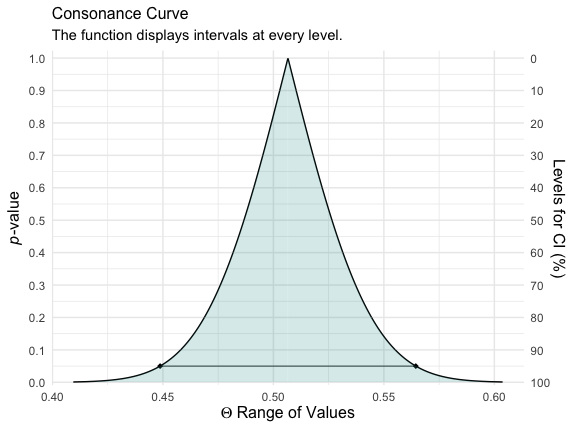ggcurve(df[], nullvalue = FALSE)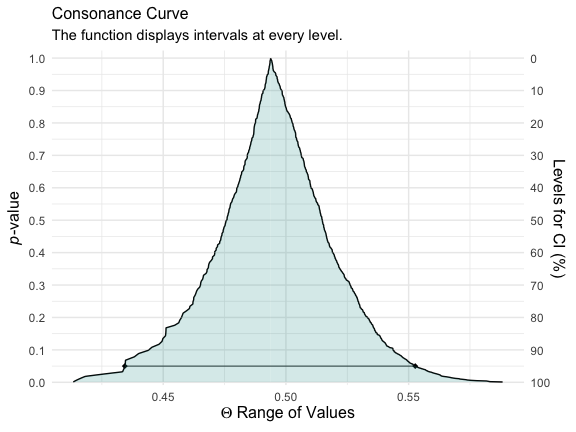We can compare the functions to see how well the bootstrap approximations match up

plot_compare(df[], df[])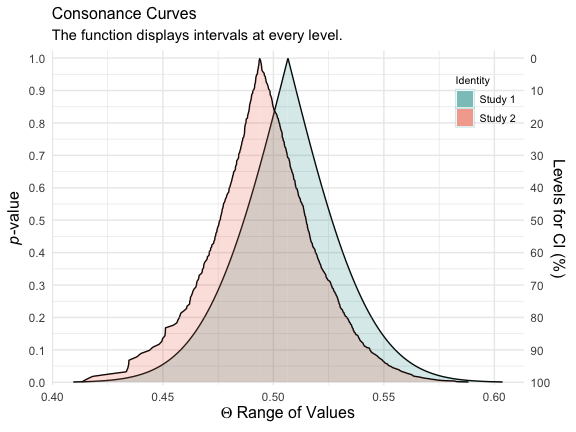We can also look at the density function

ggcurve(df[], type = "cd", nullvalue = FALSE)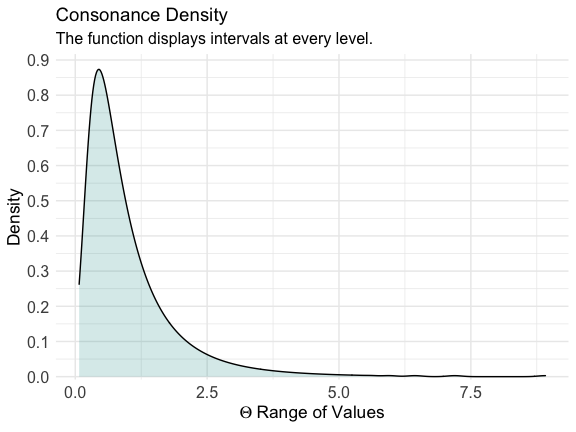That concludes our demonstration of the bootstrap method to approximate consonance functions.